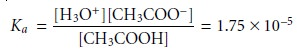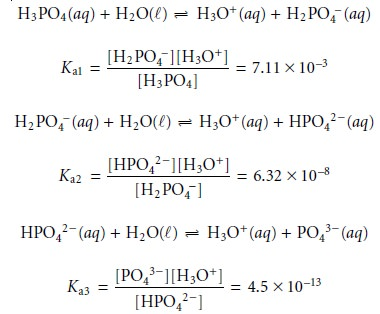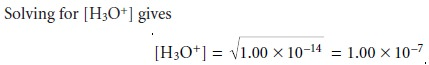Home | | Modern Analytical Chemistry | Equilibrium Constants for Acidâ€“Base Reactions

# Equilibrium Constants for Acidâ€“Base Reactions

When an acid and a base react, the products are a new acid and base.

Acidâ€“Base Reactions

A useful definition of acids and bases is that independently introduced by Jo- hannes BrĂ¸nsted (1879â€“1947) and Thomas Lowry (1874â€“1936) in 1923. In the BrĂ¸nsted-Lowry definition, acids are proton donors, and bases are proton accep- tors. Note that these definitions are interrelated. Defining a base as a proton accep- tor means an acid must be available to provide the proton. For example, in reac- tion 6.7 acetic acid, CH3COOH, donates a proton to ammonia, NH3, which serves as the base.

CH3COOH(aq)+ NH3(aq) < = = = = > CH3COOâ€“(aq)+ NH4+(aq)            6.7

 4

When an acid and a base react, the products are a new acid and base. For exam- ple, the acetate ion, CH3COOâ€“, in reaction 6.7 is a base that reacts with the acidic ammonium ion, NH4+, to produce acetic acid and ammonia. We call the acetate ion the conjugate base of acetic acid, and the ammonium ion is the conjugate acid of ammonia.

## Strong and Weak Acids

The reaction of an acid with its solvent (typically water) is called an acid dissociation reaction. Acids are divided into two categories based on the ease with which they can donate protons to the solvent. Strong acids, such as HCl, almost completely transfer their protons to the solvent molecules.

HCl(aq)+ H2O(l) â†’ H3O+(aq)+ Clâ€“(aq)

In this reaction H2O serves as the base. The hydronium ion, H3O+, is the conju- gate acid of H2O, and the chloride ion is the conjugate base of HCl. It is the hy- dronium ion that is the acidic species in solution, and its concentration deter- mines the acidity of the resulting solution. We have chosen to use a single arrow (â†’) in place of the double arrows (t) to indicate that we treat HCl as if it were completely dissociated in aqueous solutions. A solution of 0.10 M HCl is effec- tively 0.10 M in H3O+ and 0.10 M in Clâ€“. In aqueous solutions, the common strong acids are hydrochloric acid (HCl), hydroiodic acid (HI), hydrobromic acid (HBr), nitric acid (HNO3), perchloric acid (HClO4), and the first proton of sulfu- ric acid (H2SO4).

Weak acids, of which aqueous acetic acid is one example, cannot completely donate their acidic protons to the solvent. Instead, most of the acid remains undis- sociated, with only a small fraction present as the conjugate base.

CH3COOH(aq)+ H2O(l) < = = = = > H3O+(aq)+ CH3COOâ€“(aq)

The equilibrium constant for this reaction is called an acid dissociation constant,

Ka, and is written asNote that the concentration of H2O is omitted from the Ka expression because its value is so large that it is unaffected by the dissociation reaction.* The magnitude of Ka provides information about the relative strength of a weak acid, with a smaller Ka corresponding to a weaker acid. The ammonium ion, for example, with a Ka of 5.70 x 10â€“10, is a weaker acid than acetic acid.

Monoprotic weak acids, such as acetic acid, have only a single acidic proton and a single acid dissociation constant. Some acids, such as phosphoric acid, can donate more than one proton and are called polyprotic weak acids. Polyprotic acids are described by a series of acid dissociation steps, each characterized by it own acid dissociation constant. Phosphoric acid, for example, has three acid dissociation re- actions and acid dissociation constants.The
decrease in the acid dissociation constant from Ka1 to Ka3 tells us that each suc- cessive proton is harder to remove. Consequently, H3PO4 is a stronger acid than H2PO4â€“, and H PO4â€“ is a stronger acid than HPO42â€“.

## Strong and Weak Bases

Just as the acidity of an aqueous solution is a measure of the concentration of the hydronium ion, H3O+, the basicity of an aqueous solution is a measure of the concentration of the hydroxide ion, OHâ€“. The most common example of a strong base is an alkali metal hydroxide, such as sodium hydroxide, which completely dissociates to produce the hydroxide ion.

NaOH(aq) â†’ Na+(aq)+ OHâ€“(aq)

Weak bases only partially accept protons from the solvent and are characterized by a base dissociation constant, Kb. For example, the base dissociation reaction and base dissociation constant for the acetate ion arePolyprotic bases, like polyprotic acids, also have more than one base dissociation re- action and base dissociation constant.

 3

## Amphiprotic Species

Some species can behave as either an acid or a base. For ex- ample, the following two reactions show the chemical reactivity of the bicarbonate ion, HCO3 â€“, in water.A species that can serve as both a proton donor and a proton acceptor is called am- phiprotic. Whether an amphiprotic species behaves as an acid or as a base depends on the equilibrium constants for the two competing reactions. For bicarbonate, the acid dissociation constant for reaction 6.8

Ka2 = 4.69 x 10â€“11

is smaller than the base dissociation constant for reaction 6.9.

Kb2 = 2.25 x 10â€“8

 3

Since bicarbonate is a stronger base than it is an acid (kb2 > ka2), we expect that aqueous solutions of HCO3â€“ will be basic.

## Dissociation of Water

Water is an amphiprotic solvent in that it can serve as an acid or a base. An interesting feature of an amphiprotic solvent is that it is capable of reacting with itself as an acid and a base.The equilibrium constant for this reaction is called waterâ€™s dissociation con- stant, Kw,which has a value of 1.0000 x 10â€“14 at a temperature of 24 Â°C. The value of Kw varies substantially with temperature. For example, at 20 Â°C, Kw is 6.809 x 10â€“15, but at 30 Â°C Kw is 1.469 x 10â€“14. At the standard state temperature of 25 Â°C, Kw is 1.008 x 10â€“14, which is sufficiently close to 1.00 x 10â€“14 that the latter value can be used with negligible error.

## The pH Scale

An important consequence of equation 6.10 is that the concentra- tions of H3O+ and OHâ€“ are related. If we know [H3O+] for a solution, then [OHâ€“] can be calculated using equation 6.10.Equation 6.10 also allows us to develop a pH scale that indicates the acidity of a so- lution. When the concentrations of H3O+ and OHâ€“ are equal, a solution is neither acidic nor basic; that is, the solution is neutral. Letting

[H3O+] = [OHâ€“]

and substituting into equation 6.10 leaves us with

Kw = [H3O+]2 = 1.00 x 10â€“14A neutral solution has a hydronium ion concentration of 1.00 x 10â€“7 M and a pH of 7.00.* For a solution to be acidic, the concentration of H3O+ must be greater than that for OHâ€“, orThe pH of an acidic solution, therefore, must be less than 7.00. A basic solution, on the other hand, will have a pH greater than 7.00. Figure 6.3 shows the pH scale along with pH values for some representative solutions.## Tabulating Values for Ka and Kb

A useful observation about acids and bases is that the strength of a base is inversely proportional to the strength of its conjugate acid. Consider, for example, the dissociation reactions of acetic acid and acetate.The equilibrium constant for equation 6.13 is Kw. Since equation 6.13 is obtained by adding together reactions 6.11 and 6.12, Kw may also be expressed as the product of Ka for CH3COOH and Kb for CH3COOâ€“. Thus, for a weak acid, HA, and its con- jugate weak base, Aâ€“,This relationship between Ka and Kb simplifies the tabulation of acid and base dis- sociation constants. Acid dissociation constants for a variety of weak acids are listed in Appendix 3B. The corresponding values of Kb for their conjugate weak bases are determined using equation 6.14.Study Material, Lecturing Notes, Assignment, Reference, Wiki description explanation, brief detail
Modern Analytical Chemistry: Equilibrium Chemistry : Equilibrium Constants for Acidâ€“Base Reactions |